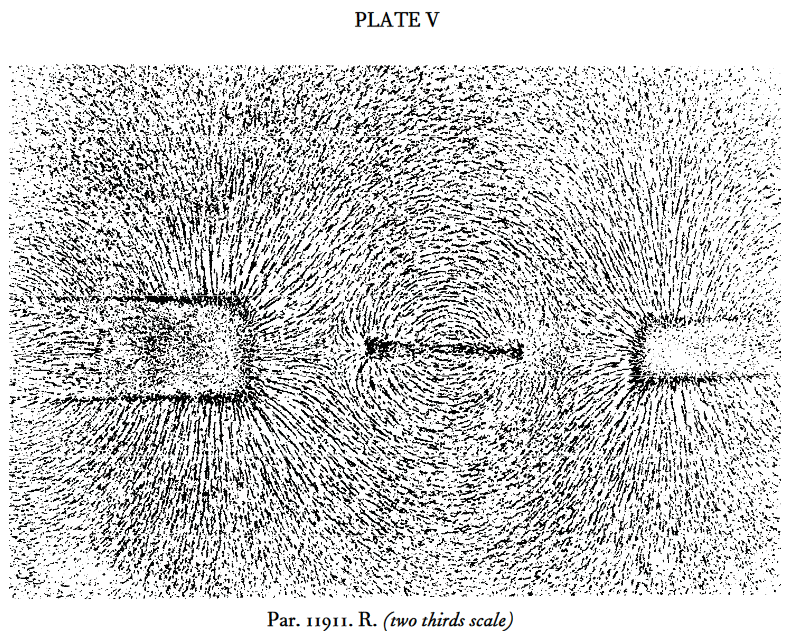# nLab flux

Contents

## Surveys, textbooks and lecture notes

#### Differential cohomology

differential cohomology

## Application to gauge theory

#### $\infty$-Chern-Weil theory

∞-Chern-Weil theory

∞-Chern-Simons theory

∞-Wess-Zumino-Witten theory

# Contents

## Definition

In gauge theory of higher abelian Yang-Mills theory type, where field configurations on some manifold $X$ are circle n-bundles with connection $\nabla$, the magnetic flux of the field configuration through a $(n+1)$-dimensional closed manifold $\Sigma \hookrightarrow X$ is

$\Phi_\sigma(\nabla) \;=\; \textstyle{\int}_\Sigma F_\nabla \,,$

where $F_\nabla$ is the curvature $(n+1)$-form.

(1)

The classical example is magnetic field-flux, originally observed by Michael Faraday by bringing iron filings into the magnetic field of rod magnets:from Faraday’s diary of experimental investigation, vol VI, entry from 11th Dec. 1851; reproduced in Martin (2009) (p. 311 here)

## Examples

### Magnetic flux quantization in type II superconductors

Due to the Meissner-Ochsenfeld effect, a superconductor placed in a sufficiently small external magnetic field $H_{ext} \lt H_{c_1}$ (aligned along some axis) “expels” that field , making the total magnetic field in the bulk of the superconductor vanish. However, as the ambient magnetic field exceeds a critical value $H_{c_1}$, this behaviour changes:

• for type I superconductors, the superconducting state simply breaks down as $H_{ext} \gt H_{c_1}$ and the ambient magnetic field fully penetrates the material as for any normal conductor;

• for type II superconductors the superconducting state eventually also breaks down as $H_{ext} \gt H_{c_2} \gt H_{c_1}$, but there is an intermediate parameter region $H_{c_1} \lt H_{ext} \lt H_{c_2}$ where both regimes mix:

In this mixed regime, a finite number of elementary units of magnetic flux enter the superconductor, carried by little flux tubes inside vortices of electric currents: vortex strings (about a micron in diameter, e.g. Chapman 00, p. 559). Each vortex core carries one unit of magnetic flux – also called a fluxon – while at some small finite distance away from all vortices, the bulk magnetic flux in the superconductor still vanishes (mathematically: it vanishes at infinity, cf. eg. Chapman 2009 (2.31), Timm 2020 (6.101)) :

(2)

At sufficiently large density these vortices arrange into hexagonal patterns, first described by Abrikosov 57, whence also known as Abrikosov vortices.

(3)

This flux quantization in type II superconductors is traditionally explained via the effective field theory provided by the Landau-Ginzburg model; the derivation may be found reviewed in Chapman 00 (Section 2, culminating in (2.33)).

But, as indicated a little more explicitly in Alvarez-Gaumé 98 (Section IV.B, culminating below IV.11), the flux quantization as such is mathematically a direct consequence of the global topological nature of the electromagnetic field, the argument being the direct 2-dimensional analog of the quantization of instantons in QCD in 4d (see also at SU(2)-Instantons – From the correct maths to the traditional physics story) and in fact is but a slight variation of the argument for Dirac charge quantization of magnetic monopoles:

Namely, the electromagnetic field is a connection on a circle bundle, and hence the cohomology classifying space for the topological class of the electromagnetic field is the classifying space $B \mathrm{U}(1)$ of the circle group, which, being an Eilenberg-MacLane space $K(\mathbb{Z},2)$, has second homotopy group the integers:

$\pi_2(B \mathrm{U}(1)) \;\simeq\; \big\{ S^2 \to B \mathrm{U}(1)\big\}_{\big/hmpty} \;\simeq\; \mathbb{Z} \,.$

This implies that on every spacetime which looks, up to homotopy equivalence, like a 2-sphere to the electromagnetic field, the magnetic flux is identified with an element in the group of integers, hence is quantized in integral multiples of some unit flux.

For the case of a (hypothetical) magnetic monopole (e.g. a magnetically charged black hole), it is the spacetime around the monopole (the complement of its worldline $\mathbb{R}^{0,1}$ in the ambient (asymtptotically) Minkowski spacetime $\mathbb{R}^{3,1}$) which has the homotopy type of a 2-sphere $\mathbb{R}^{3,1} \setminus \mathbb{R}^{0,1} \,\simeq\, \mathbb{R}^{0,1} \times \mathbb{R}_{rad} \times S^2$; this implies the Dirac charge quantization of the magnetic monopole‘s magnetic charge:

For the case of the type II superconductor it is instead the transversal vanishing at infinity of the magnetic field (i.e. the Meissner-Ochsenfeld effect away from the vortices) which implies that the classifying map of the electromagnetic field sees not the full transversal Euclidean plane $\mathbb{R}^2$ but its one-point compactification $\big( \mathbb{R}^2 \big)^{cpt} \,\simeq\, S^2$, which introduces an effective 2-sphere topology onto spacetime (same as the 3-sphere in the discussion of Skyrmions and the 4-sphere in the discussion of instantons): $\mathbb{R}^{1,1} \times \big( \mathbb{R}^2 \big)^{cpt} \,\simeq\, \mathbb{R}^{1,1} \times S^2$. This implies the superconductor’s magnetic flux quantization: